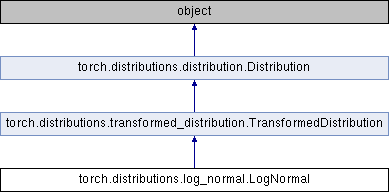Caffe2 - Python API A deep learning, cross platform ML framework
torch.distributions.log_normal.LogNormal Class Reference
Inheritance diagram for torch.distributions.log_normal.LogNormal:## Public Member Functions

def __init__ (self, loc, scale, validate_args=None)

def expand (self, batch_shape, _instance=None)

def loc (self)

def scale (self)

def mean (self)

def variance (self)

def entropy (self)Public Member Functions inherited from torch.distributions.transformed_distribution.TransformedDistribution
def __init__ (self, base_distribution, transforms, validate_args=None)

def expand (self, batch_shape, _instance=None)

def support (self)

def has_rsample (self)

def sample (self, sample_shape=torch.Size())

def rsample (self, sample_shape=torch.Size())

def log_prob (self, value)

def cdf (self, value)

def icdf (self, value)Public Member Functions inherited from torch.distributions.distribution.Distribution
def __init__ (self, batch_shape=torch.Size(), event_shape=torch.Size(), validate_args=None)

def expand (self, batch_shape, _instance=None)

def batch_shape (self)

def event_shape (self)

def arg_constraints (self)

def support (self)

def mean (self)

def variance (self)

def stddev (self)

def sample (self, sample_shape=torch.Size())

def rsample (self, sample_shape=torch.Size())

def sample_n (self, n)

def log_prob (self, value)

def cdf (self, value)

def icdf (self, value)

def enumerate_support (self, expand=True)

def entropy (self)

def perplexity (self)

def __repr__ (self)

## Static Public Attributes

arg_constraints

support

has_rsampleStatic Public Attributes inherited from torch.distributions.transformed_distribution.TransformedDistribution
arg_constraintsStatic Public Attributes inherited from torch.distributions.distribution.Distribution
has_rsample

has_enumerate_support

support

arg_constraints

## Additional Inherited MembersStatic Public Member Functions inherited from torch.distributions.distribution.Distribution
def set_default_validate_args (value)Public Attributes inherited from torch.distributions.transformed_distribution.TransformedDistribution
base_dist

transforms

## Detailed Description

Definition at line 8 of file log_normal.py.

The documentation for this class was generated from the following file: